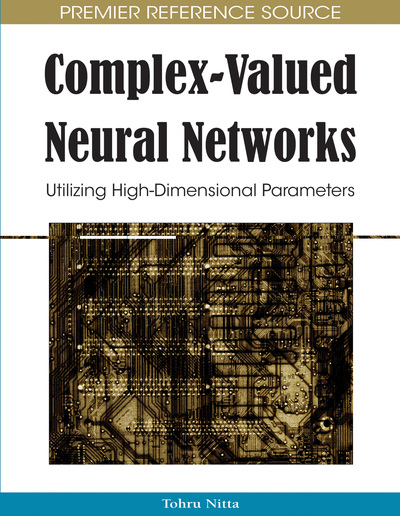# Complex-Valued Neural Network and Inverse Problems

Takehiko Ogawa (Takushoku University, Japan)
DOI: 10.4018/978-1-60566-214-5.ch002
Available
\$37.50
No Current Special Offers

## Abstract

Network inversion solves inverse problems to estimate cause from result using a multilayer neural network. The original network inversion has been applied to usual multilayer neural networks with real-valued inputs and outputs. The solution by a neural network with complex-valued inputs and outputs is necessary for general inverse problems with complex numbers. In this chapter, we introduce the complex-valued network inversion method to solve inverse problems with complex numbers. In general, difficulties attributable to the ill-posedness of inverse problems appear. Regularization is used to solve this ill-posedness by adding some conditions to the solution. In this chapter, we also explain regularization for complex-valued network inversion.
Chapter Preview
Top

## Introduction

It is necessary to solve inverse problems for estimating causes from observed results in various engineering fields. In particular, inverse problems have been studied in the field of mathematical science (Groetsch, 1993). The inverse problem determines the inner mechanisms or causes of an observed phenomenon. The cause is estimated from the fixed model and the given result in the inverse problem, while the result is determined from the given cause by using a certain fixed mathematical model in the forward problem. As a solution of inverse problems, the neural network based method has been proposed while other method such as the statistical method (Kaipio & Somersalo, 2005) and parametric method (Aster, Borchers, & Thurber, 2005) have also been studied.

The idea of inverting network mapping was proposed by Williams (1986). Then, Linden and Kindermann proposed a method of network inversion (Linden & Kindermann, 1989). Also, the algorithms and applications of network inversion are summarized by Jansen et al. (1999). In this method, inverse problems are solved by the inverse use of the input-output relation of trained multilayer neural networks. In other words, the corresponding input is estimated from the provided output via fixed weights, after finding the forward relation by network training. The direction of the input-output relation between the training and the inverse estimation is important in this method. The estimation process in multilayer neural networks is considered from the viewpoint of forward and inverse problems. The usual estimation process of multilayer neural networks provides a solution for forward problems because the network estimates the output from the input provided by the forward relation obtained in the training. On the other hand, we can solve inverse problems using multilayer neural networks that learn the forward relation by estimating the input from the given output inversely. Network inversion has been applied to actual problems; e.g., medical image processing (Valova, Kameyama, & Kosugi, 1995), robot control (Lu & Ito, 1995; Ogawa, Matsuura, & Kanada, 2005), optimization problems, and so on (Murray, Heg, & Pohlhammer, 1993; Ogawa, Jitsukawa, Kanada, Mori, & Sakata, 2002; Takeuchi & Kosugi, 1994). Moreover, the answer-in-weights scheme has been proposed to solve the difficulty of ill-posed inverse problems, as a related model of network inversion (Kosugi & Kameyama, 1993).

The original network inversion method proposed by Linden and Kindermann solves an inverse problem by using a usual multilayer neural network that handles the relation between real-valued input and output. However, a network method for complex-valued input and output is required to solve the general inverse problem whose cause and result extend to the complex domain. On the other hand, there exists an extension of the multilayer neural network to the complex domain (Benvenuto & Piazza, 1992; Hirose, 2005; Nitta, 1997). The complex-valued neural network learns the relations between complex-valued input and output in the form of complex-valued weights. This complex-valued network inversion was considered to solve inverse problems that extended to complex-valued input and output. In this method, the complex-valued input is inversely estimated from the provided complex-valued output by extending the input correction of the original network inversion method to the complex domain. Actually, the complex-valued input is estimated from the complex-valued output by giving a random input to the trained network, back-propagating the output error to the input, and correcting the input (Ogawa & Kanada, 2005a).

## Complete Chapter List

Search this Book:
Reset